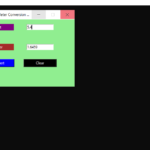## Simple Height Calculator In Python with Source Code

The Simple Height Calculator In Python is a simple project developed using Python. The project is for the user convenient, to help them convert their height from one unit to another. This project is for height calculating to figure out the heights in diff...

Type : Project

File Size : 637 bytes# Similar Projects and Reports

#### Simple Height Calculator In Python with Source Code

The Simple Height Calculator In Python is a simple project developed using Python. The project is for the user convenient, to help them convert their height from one unit to another. This project is for height calculating to figure out the heights in diff...

#### SIMPLE CALCULATOR IN PYTHON WITH SOURCE CODE

The Simple Calculator In Python is a simple project developed using Python. The project contains the numbers, operators, and sign like in normal calculator. The user can enter any number or can just click on the numbers they want in the calculations. Henc...

#### CALCULATOR IN PYTHON WITH SOURCE CODE

This Calculator project In Python is a simple project developed using Python. The project contains the numbers, operators, and sign like in normal calculator. So, the user can enter any number they want in the calculations. Hence, the user can use a simpl...

#### SIMPLE GROCERY PRICE CALCULATOR IN PYTHON WITH SOURCE CODE

This simple grocery price calculator project is written in Python. The project file contains a python script (grocery_app.py). This is a simple GUI based application which is very easy to understand and use. It uses Tkinter module for the GUI. Talking abo...

#### Tip Calculator In Python with Source Code

The Tip Calculator In Python is a simple project developed using Python. The project is for the user convenient, for calculating the tip. This project is an interesting project. The user can calculate the tip amount that they can get from a single bill pa...

#### BMI Calculator In Python With Source Code

The BMI Calculator In Python is a simple project developed using Python. The project is for the user convenient, for checking your BMI. With the BMI value, you can check whether you have a healthy weight or not. The project file contains a python script ...